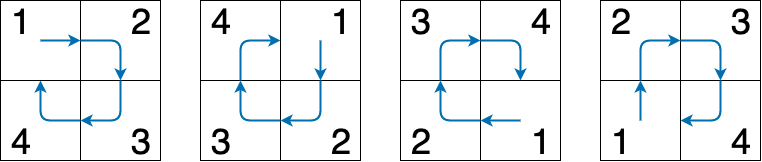시간 제한메모리 제한제출정답맞힌 사람정답 비율
1 초 (추가 시간 없음) 256 MB207735.000%

## 문제

Lee got a ticket to the Google Developer Day. When he came to the exhibition hall, he found that the booths were located in a matrix of size $n \times m$.

Unfortunately, Lee had badly sprained his left ankle a week before the GDD when playing basketball. As a result, he couldn't wander freely as he wanted. His ankle would get hurt if he tried to turn left. Lee also didn't want to make a big right turn by turning right multiple times, which would make him look stupid.

Lee wanted to visit each booth exactly once. Given his ankle situation, he made some rules visiting the booths. He could start with any booth in the exhibition hall, and choose an initial direction. Then each move would have to follow one of the possible moves:

1. Go straight to visit the next booth.
2. Turn right once and then go straight to visit the next booth.

You are a friend of Lee and you have the good habit of helping him. Can you find out the number of different ways to visit each booth exactly once? Two ways are considered different if and only if the visiting orders in these two ways vary.

## 입력

The first line of the input gives the number of test cases, $T$ ($1 \le T \le 100$). $T$ test cases follow.

For each test case, the first and only line contains two integers $n$ and $m$ ($1 \le n, m \le 100$), the number of rows and the number of columns of the matrix, respectively.

## 출력

For each test case, output one line containing "Case #x: y", where x is the test case number (starting from $1$), and y is the number of different ways modulo ($10^9+7$).

## 예제 입력 1

1
2 2


## 예제 출력 1

Case #1: 4


## 힌트

Here are the 4 different ways for $n = 2, m = 2$.